# BIOLPHYS 438 Logistics Ch 5 Animals in Motion

• Slides: 22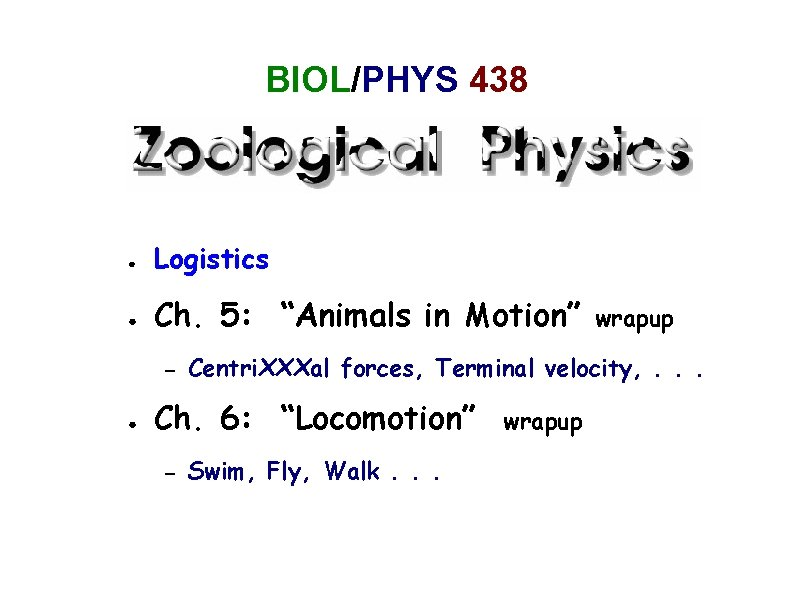BIOL/PHYS 438 ● Logistics ● Ch. 5: “Animals in Motion” – ● Centri. XXXal forces, Terminal velocity, . . . Ch. 6: “Locomotion” – wrapup Swim, Fly, Walk. . . wrapup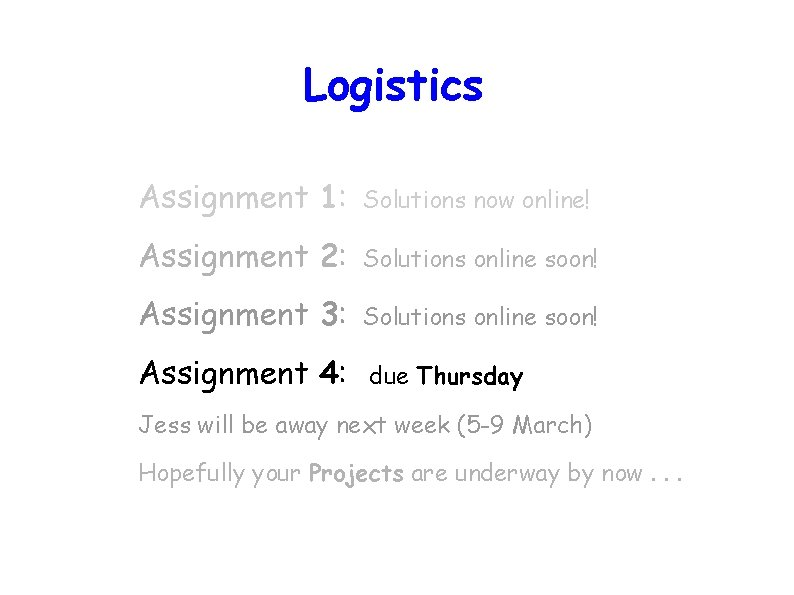Logistics Assignment 1: Solutions now online! Assignment 2: Solutions online soon! Assignment 3: Solutions online soon! Assignment 4: due Thursday Jess will be away next week (5 -9 March) Hopefully your Projects are underway by now. . .Circular Motion review Clarification: centripetal vs. “centrifugal” forces: In order for a particle of mass in a circle of radius r about a center C, it must be subjected to a m • m to move at a constant speed v centripetal (“toward the center”) force of magnitude F' • C F = m v 2/ r = m r ω 2 (where ω ≡ d /dt ) in order to cause the centripetal acceleration a = v 2/r = r ω 2 implicit in uniform circular motion. This implies an equal and opposite centrifugal force F' acting on the center C (not on the particle!).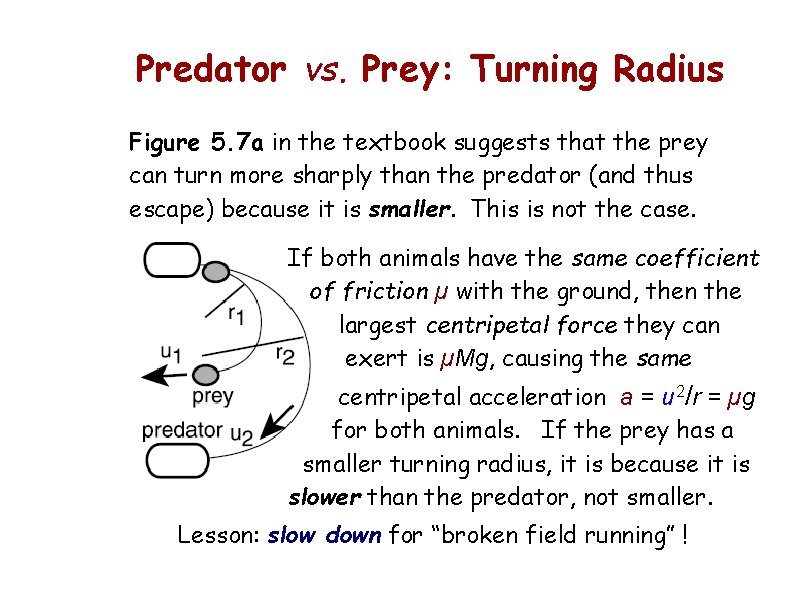Predator vs. Prey: Turning Radius Figure 5. 7 a in the textbook suggests that the prey can turn more sharply than the predator (and thus escape) because it is smaller. This is not the case. If both animals have the same coefficient of friction µ with the ground, then the largest centripetal force they can exert is µMg, causing the same centripetal acceleration a = u 2/r = µg for both animals. If the prey has a smaller turning radius, it is because it is slower than the predator, not smaller. Lesson: slow down for “broken field running” !Crane Stance A karate master stands in position A. How can she get to position C in one move without bending her knees or sliding her feet on the floor ? A CAnswer: first she quickly lifts her arms, raising her centre of gravity to the desired level and giving it a small upward momentum; then she abruptly reverses the motion and lowers her arms, lifting the rest of her body so that she can move her legs inward freely. Extra normal forces A Normal forces = 0 B C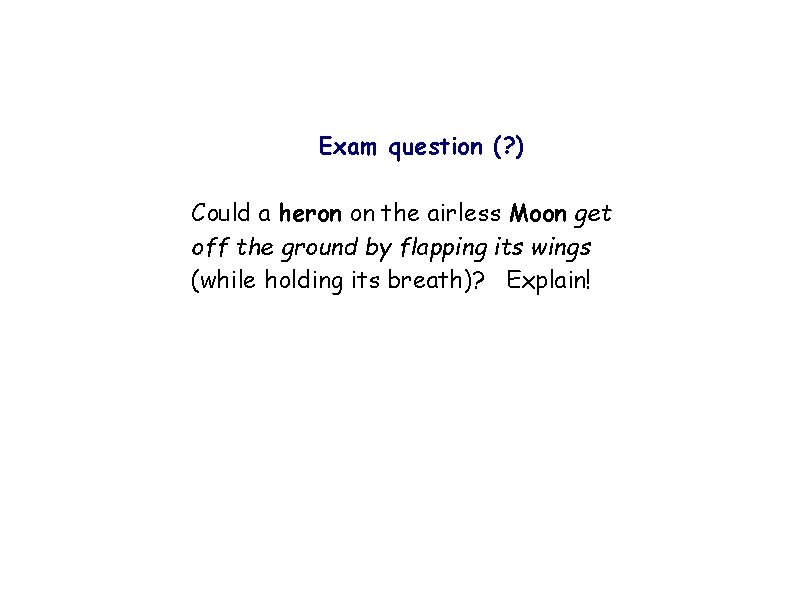Exam question (? ) Could a heron on the airless Moon get off the ground by flapping its wings (while holding its breath)? Explain!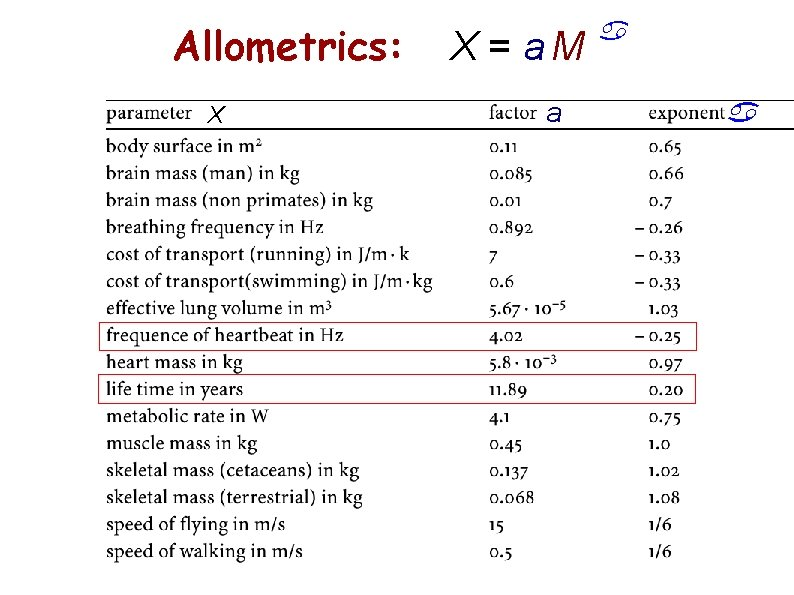Allometrics: X X = a. M a a aHeartbeat Quota: Nhb = 4. 02 11. 89 (365 24 60 60) M = 1. 5 10 9 M – 0. 05 more or less independent of M. – 0. 05Terminal Velocity -- when Sphere: 4 Mg = Fdrag /3 p R 3 r g = Fdrag = 6 p R hu + ½ p R 2 CD r u 2 For Re > 100, CD ~ 1; for Re < 100, Fh « Fs so it does no harm to set CD = 1. Then we can solve a quadratic equation for u: FStokes Fhydrodynamic Recr ≈ 100 (external flow) vs. ≈ 2300 (pipes) u = (6 h/R r) (√ 1 + (R 3 r g /27 h 2 ) − 1) Re = 2 u R / n = 2 u R r / h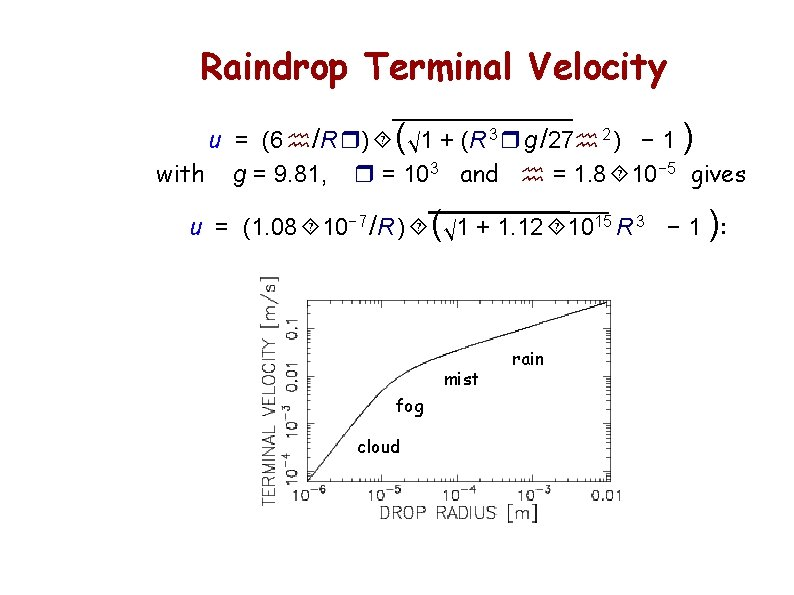Raindrop Terminal Velocity u = (6 h/R r) (√ 1 + (R 3 r g /27 h 2 ) − 1 ) with g = 9. 81, r = 103 and h = 1. 8 10− 5 gives u = (1. 08 10− 7 /R ) (√ 1 + 1. 12 1015 R 3 − 1 ): mist fog cloud rainJet Propulsion Bernoulli: Δp = ½ r u 2 mass flow: J = p r 2 r u Thrust: F = p r 2 Δp = ½ p r 2 r u 2 = J u = J 2 / p r 2 r There's a factor of 2 discrepancy. .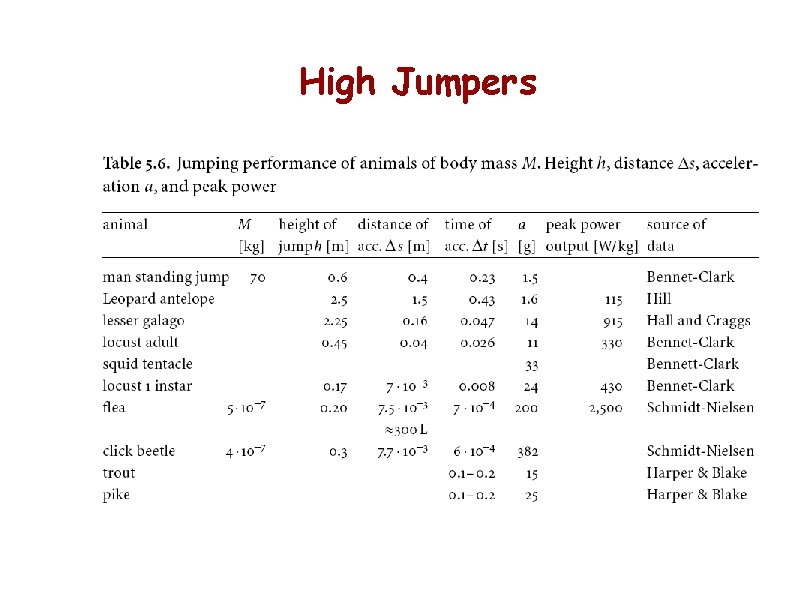High JumpersAllometrics: X X = a. M a a aEnergy per Distance per Body Mass Cost of Transport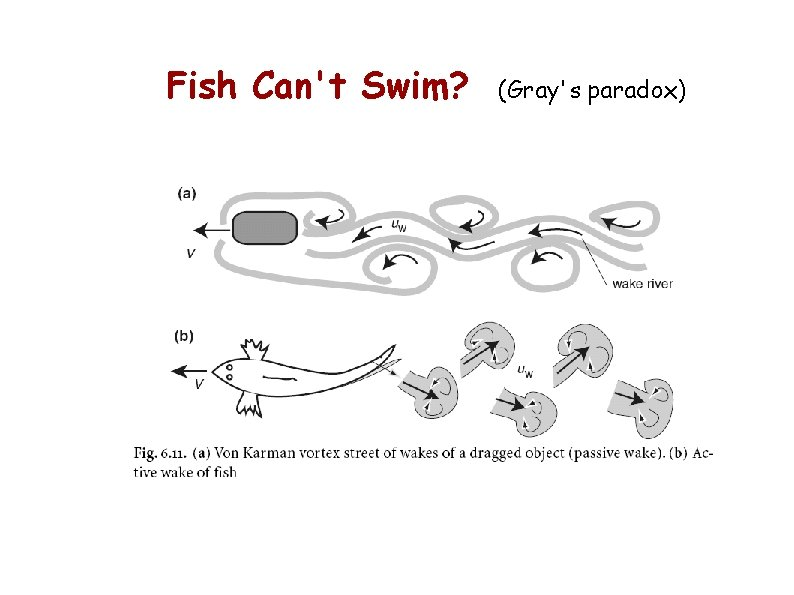Kicking off the Eddies (what goes around, comes around)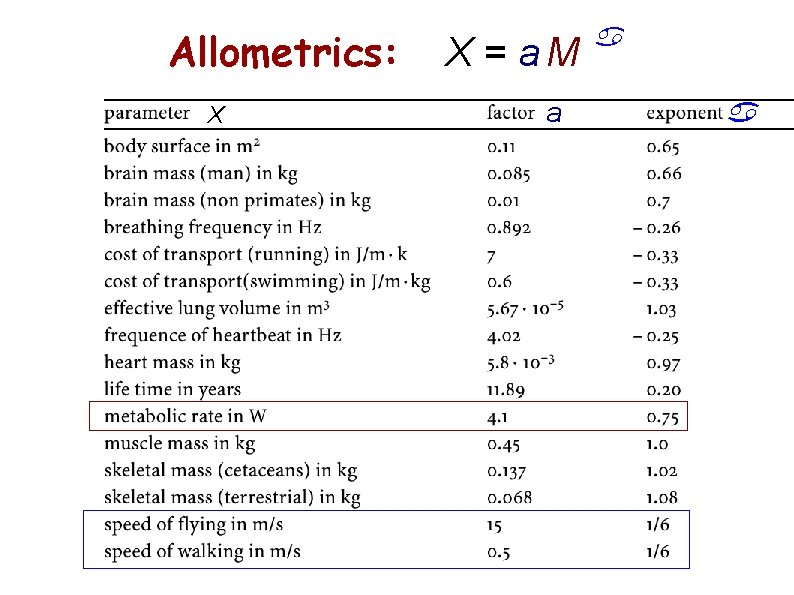Allometrics: X X = a. M a a aSwimmers Speed Usw ≈ 0. 5 M 0. 19 Length L large ≈ 1. 5 M 0. 43Fliers Speed U ≈ 15 M 1/6 Metabolic velocity Um ≈ 40 M – 1/4Runners Endurance running speed Uer ≈ Cr M – 0. 023 ≈ const.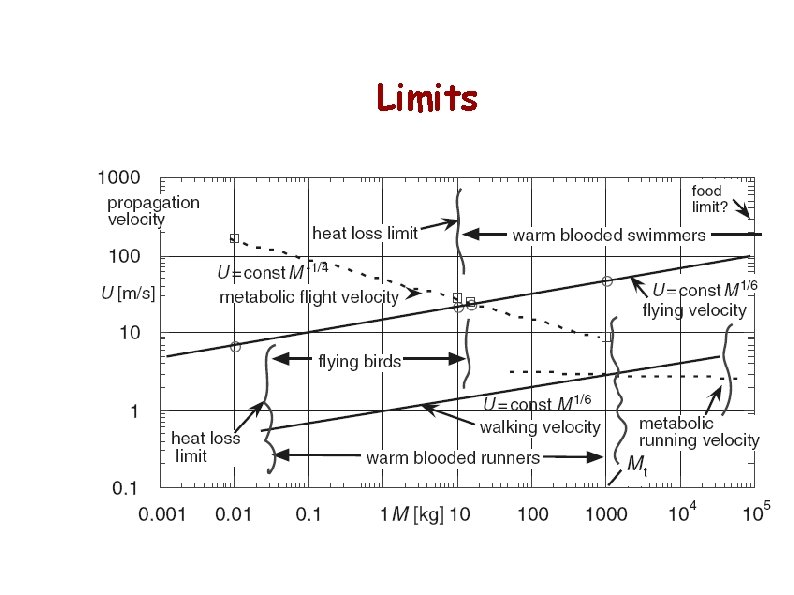Limits